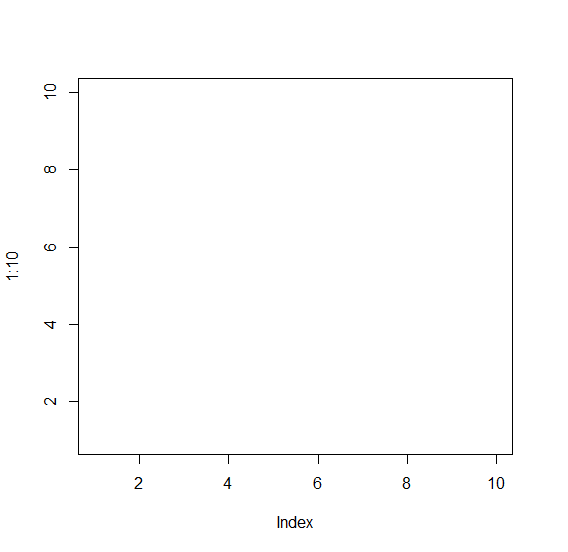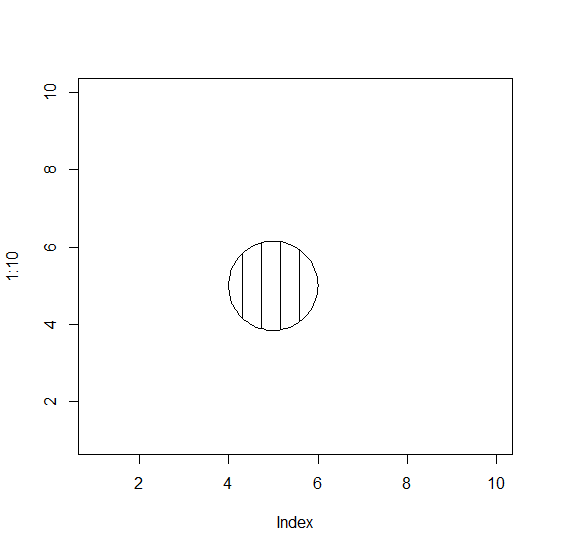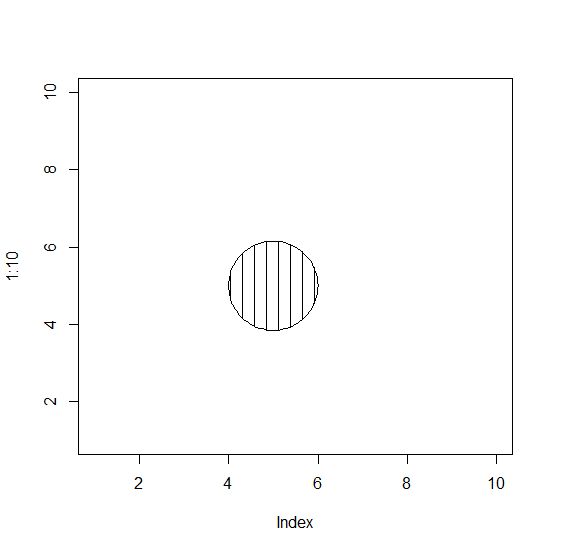# How to create a circle with vertical lines in R?

We can create a circle in R by using draw.circle function of plotrix package and if we want to have vertical lines inside the circle then density and angle arguments will be used. The density argument will create the lines and angle argument will set the direction of those lines.

For vertical lines the angle will be 270 and the number of lines depends on the value of density argument.

Check out the below Examples to understand how different values of density create lines inside the circle.

## Example

To create a circle in R, use the code given below −

plot(1:10,type="n")

## Output

If you execute the above given snippet, it generates the following Output −To create a circle in R, add the following code to the above snippet −

plot(1:10,type="n")
draw.circle(5,5,1,density=5,angle=270)

## Output

If you execute all the above given snippets as a single program, it generates the following Output −To create a circle in R, add the following code to the above snippet −

plot(1:10,type="n")
draw.circle(5,5,1,density=8,angle=270)

## Output

If you execute all the above given snippets as a single program, it generates the following Output −